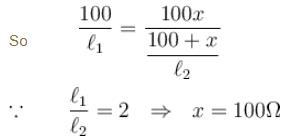Courses

# Current Electricity MCQ Level - 2

## 10 Questions MCQ Test Basic Physics for IIT JAM | Current Electricity MCQ Level - 2

Description
This mock test of Current Electricity MCQ Level - 2 for Physics helps you for every Physics entrance exam. This contains 10 Multiple Choice Questions for Physics Current Electricity MCQ Level - 2 (mcq) to study with solutions a complete question bank. The solved questions answers in this Current Electricity MCQ Level - 2 quiz give you a good mix of easy questions and tough questions. Physics students definitely take this Current Electricity MCQ Level - 2 exercise for a better result in the exam. You can find other Current Electricity MCQ Level - 2 extra questions, long questions & short questions for Physics on EduRev as well by searching above.
QUESTION: 1

### 50V  battery is supplying current of 10 amp when connected to a resistor. If the efficiency of battery at this current is 25%. Then internal resistance of battery is :

Solution:

50 = 10[R + r]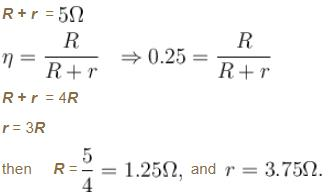QUESTION: 2

### A cell of emf E having an internal resistance ‘r’  is connected to an external resistance R. The potential difference ‘V’ across the resistance R varies with R as shown by the curve :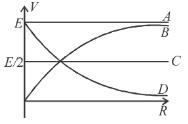Solution: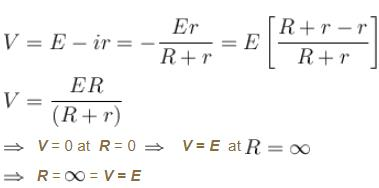QUESTION: 3

### ‘n’  identical light bulbs, each designed to draw P power from a certain voltage supply are joined in series and that combination is connected across that supply. The power consumed by one bulb will be :

Solution:

Voltage across each bulb will be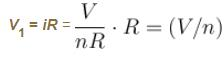so, power developed by each bulb = iV1 =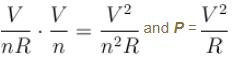so, power consumed by one bulb =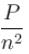QUESTION: 4

A copper sphere of 10cm  diameter is lowered into a water filled hemispherical copper vessel of 20cm  diameter so that the sphere and the vessel becomes concentric. Electrical conductivity of water of  σ = 10-3(Ωm)-1. The electrical resistance between the sphere and the vessel is :

Solution:

The arrangement is shown in figure. Consider the hemispherical shell of radius r and thickness dr as shown.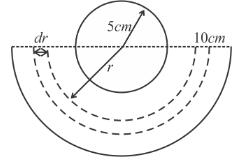QUESTION: 5

When a galvanometer is shunted with a 4Ω resistance, the deflection is reduced to one-fifth. If the galvanometer is further shunted with a 2Ω wire, the further reduction (find the ratio of decrease in current to the previous current) in the deflection will be (the main current remains the same).

Solution:

Case I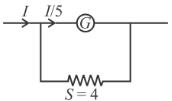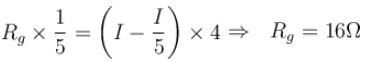Case II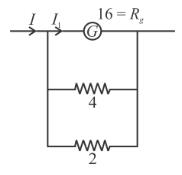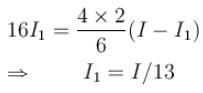so decrease in current to previous current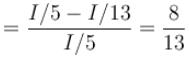The correct answer is: (8/13) of the deflection when shunted with 4Ω only

QUESTION: 6

In the circuit shown, the value of R in ohm that will result in no current through the 30V battery, is:]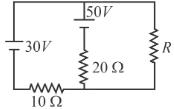Solution: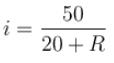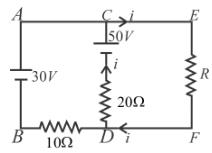Potential drop across R = Potential drop across AB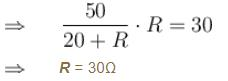QUESTION: 7

In the shown wire frame, each side of a square (the smallest square) has a resistance R. The equivalent resistance of the circuit between the points A and B is :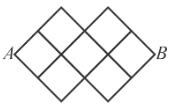Solution: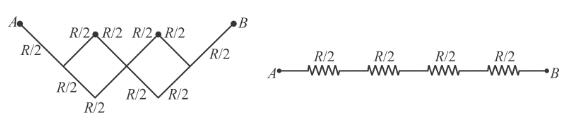Hence equivalent resistance between A  and B  is 2R.

QUESTION: 8

Two long coaxial and conducting cylinders of radius a and b are separated by a material of conductivity σ and a constant potential difference V  is maintained between them, by a battery. Then the current, per unit length of the cylinder flowing from one cylinder the other is:

Solution: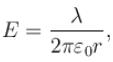where is the linear charge density on the inner cylinder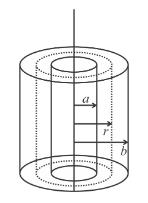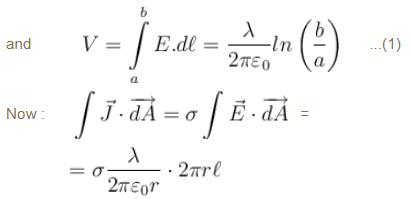Current per unit length will be :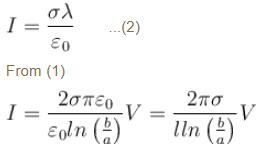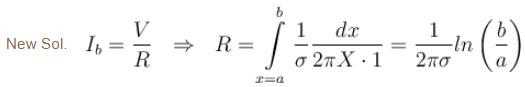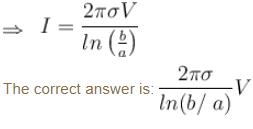QUESTION: 9

A cylindrical solid of length and radius a  is having varying resistivity given by  ρ = ρ0x  where ρ0 is a positive constant and x is measured from left end of solid. The cell shown in the figure is having emf V and negligible internal resistance. The electric field as a function of x is best described by :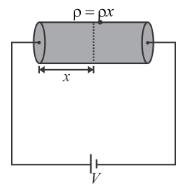Solution:

Consider an elemental part of solid at a distance  x  from left end of width dx.
Resistance of this elemental part is,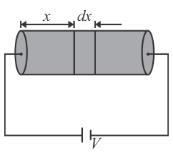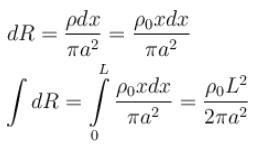Current through cylinder is,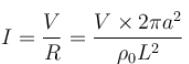Potential drop across element is,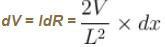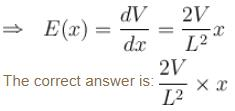QUESTION: 10

In a practical wheat stone bridge circuit as shown, when one more resistance of 100Ω is connected is parallel with unknown resistance x, then ratio ℓ1/ℓ2 become 2, ℓ1 is balance length. AB is a uniform wire. Then value of x  must be :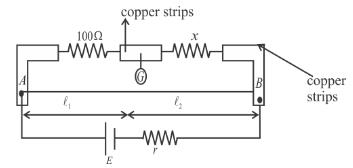Solution: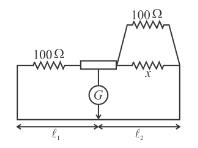∵  wheat stone bridge is in balanced condition